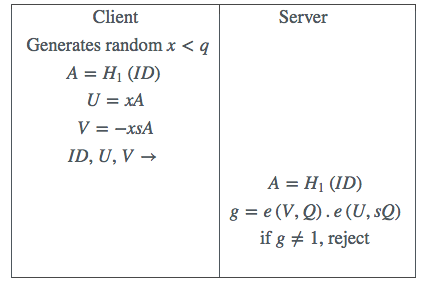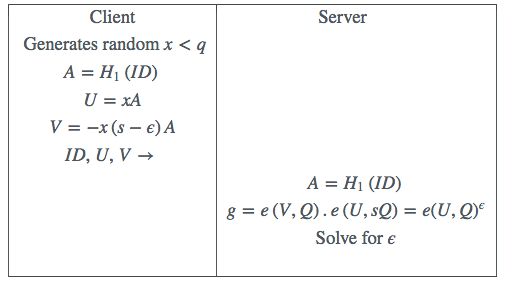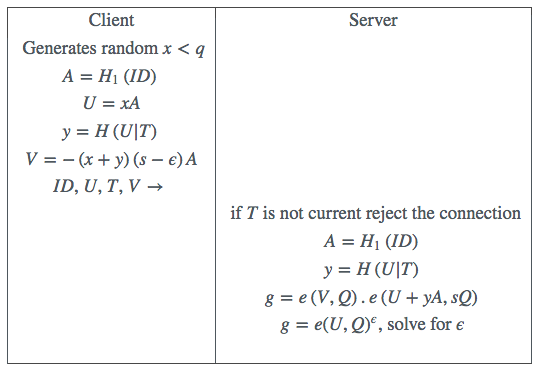# The Carnac protocol – or how to read the contents of a sealed envelope

##### Dr Michael Scott

Johnny Carson as long time host of the Tonight show often appeared in the spoof role of Carnac the Magnificent, a mentalist who could magically read the contents of a sealed envelope. This is in fact a well known stock-in-trade trick of the mentalist’s craft, known as “billet reading”. Here we propose a cryptographic solution to the problem of billet reading, apparently allowing a ciphertext to be decrypted without direct knowledge of the ciphertext, and present both a compelling use case and a practical implementation.

### 1 Introduction

A credit card owner, a customer of a credit card company, wants to carry his credit card number (CCN) around on his mobile phone, but stored in an encrypted form (lest his mobile phone should be stolen), that is tied to his identity. We call this the Ciphertext. However no encryption or decryption keys are available to the owner. So although the owner may know his own credit card details, the owner cannot either encrypt another’s details, or indeed decrypt his own Ciphertext.

At some point the owner decides to make a purchase from a merchant. The merchant knows nothing about the owner or their credit card, and has nothing stored locally related to either. Ideally the credit card owner wants to enter into a protocol with the merchant using the Ciphertext such that (a) the merchant learns nothing about the Ciphertext (lest a dishonest merchant should use the Ciphertext for their own purchases), but nonetheless (b) the merchant is able to determine the credit card number and the associated authenticated identity of its owner.

An immediate objection might be that in this scenario a stolen Ciphertext is as good as a CCN, as it can clearly be used to make purchases from the merchant. Later we shall show how, in a practical implementation, the Ciphertext can be adequately protected just using a short PIN number. Using a PIN number to protect credit card transactions is a well known and trusted mechanism, and the same familiar PIN can be used here.

Clearly a trusted authority (TA) must be involved, probably belonging to the credit card company, as otherwise there is no way to encrypt the CCN. Assume that this TA is known to both the customer and the merchant. The TA has its own master key $s$. The customer approaches the TA who encrypts the CCN using the encryption key $s$ to create the Ciphertext ${E}_{s}\left(CCN\right)$, which is given to the customer. Note that it is not essential that the customer actually knows their own CCN. The TA also issues to the merchant a value ${D}_{s}$, derived from $s$, but from which $s$ cannot be extracted.

The idea now is that the customer should be able to enter into a protocol with the merchant, using ${E}_{s}\left(CCN\right)$, such that the merchant ends up knowing CCN and the identity of its owner, but in the process learns nothing about ${E}_{s}\left(CCN\right)$. By analogy the customer is able to magically determine CCN without touching the “sealed envelope” that is ${E}_{s}\left(CCN\right)$. The trick is (and there has to be a trick!) is that the mentalist/merchant has in their possession the apparently unrelated secret ${D}_{s}$.

For the purposes of this paper we assume that a credit card number consists of 5 blocks of 4 decimal digits each.

### 2 A simple authentication protocol

To realise our solution, we will use pairing-based cryptography. To be concrete we assume the use of a BN elliptic curve  at the AES-128 level of security. This is (and for our purposes must be) a type-3 pairing , where $e:{G}_{1}×{G}_{2}\to {G}_{T}$. The groups ${G}_{1}$, ${G}_{2}$ and ${G}_{T}$ are all of the same prime order $q$. We make standard pairing-based security assumptions, including the XDH assumption that the decisional Diffie-Hellman problem is hard in ${G}_{1}$.

First consider a simple protocol, in which a client tries to authenticate its identity to a server. We assume that the server is authenticated to the client in a standard way, perhaps using the well known SSL protocol. Here $ID$ is the client identity and ${H}_{1}\left(.\right)$ is a hash function which hashes its input to a point on ${G}_{1}$.

This protocol attempts to prove in zero knowledge to the server that the owner of the claimed identity $ID$ is in possession of the value $sA$ issued to it by the TA, where $A={H}_{1}\left(ID\right)$. The same TA has issued the secret $sQ\in {G}_{2}$ to the server, where $Q$ is a fixed public point in ${G}_{2}$. Correctness follows immediately from the well known bilinearity property of the pairing. See Table 1.Table 1: A simple authentication protocol

As an authentication protocol this has some obvious deficiencies (for example it is subject to a simple replay attack), but for the moment we will ignore them. Next we introduce a small error $ϵ$ into the client’s secret $sA$. See Table 2.Table 2: The subliminal channel

Observe now that the value of $g$ as calculated on the server side can be used to recover $ϵ$. Strictly speaking as an authentication protocol we might consider that the authentication has failed. Or we may permit a small deviation $ϵ$ and judge the authentication a success. In either case we claim that the successful transmission of $ϵ$ represents a secure narrow-band subliminal channel  whereby a short secret $ϵ$ can be communicated from the client to the server.

For the server to find $ϵ$ requires the calculation of a pairing and the solution of a discrete logarithm problem in ${G}_{T}$. The appropriate algorithm is the method of Pollard’s Kangaroos . This is a “square root” algorithm, which means that for a 4-digit $ϵ$ usually only a few hundred multiplications in ${G}_{T}$ will be required to find $ϵ$, which is completely practical.

To establish the security of the subliminal channel consider a powerful passive observer who has recorded the communication and subsequently somehow captures the un-adjusted client secret $sA$. Now they are in possession of $sA$, $xA$ and $x\left(s-ϵ\right)A$. However even this does not reveal $ϵ$, as a consequence of the XDH assumption,

Finally we upgrade our protocol to use a non-interactive version of a provably secure zero-knowledge protocol for proof of identity , section 5.3, introducing a time stamp $T$ to prevent replay attacks. See Table 3, where the hash function $H\left(.\right)$ hashes its input to an element in ${F}_{q}$, and the symbol $|$ indicates simple concatenation of the inputs.Table 3: The final protocol

### 3 Our use case solution

The way forward is now quite straightforward. The TA issues to the customer whose identity is proven to be $ID$ the secrets ($s-{ϵ}_{n}$)${H}_{1}\left(ID|n\right)$ where ${ϵ}_{n}$ is $n$-th block of 4 digits of the CCN. This is done for each of the 5 blocks of the CCN, for $n=$ 1 to 5. This now constitutes our ciphertext ${E}_{s}\left(CCN\right)$. On the server side ${D}_{s}=sQ$. To communicate the CCN to the merchant’s server, we run the above protocol 5 times. In practice the 5 client to server communications can all be batched into a single blob of data, sharing the same time-stamp.

### 4 Discussion

In the particular use case considered here, the identity used in the protocol is of no particular significance, and may be a pseudonym in a predetermined format to preserve anonymity. However this identity is authenticated in the course of the protocol, and is assumed to be bound to the associated CCN in the back-office payment centre.

To protect the Ciphertext a simple idea borrowed from  is to subtract from each component of it a PIN number, so that the secrets are now ($s-{ϵ}_{n}-PIN$)${H}_{1}\left(ID|n\right)$. This PIN can then be re-inserted when the protocol is run. Clearly without the correct PIN, a valid CCN will not be received by the server.

If the merchant’s secret is stolen then clearly all captured Ciphertexts can be decrypted. However a stolen merchant secret cannot by itself be used to make purchases from honest merchants.

To remove any single point of failure, the TA can be distributed. In the simplest scenario the client can retrieve ${s}_{1}{H}_{1}\left(ID\right)$ from one TA, ${s}_{2}{H}_{1}\left(ID\right)$ from a second TA, and simply add them together to create $s{H}_{1}\left(ID\right)$, where $s={s}_{1}+{s}_{2}$. In this case the adjusted secret for our use case would be created by subtracting the ${ϵ}_{i}$ from just one of the TA master secret shares. Also the equivalent server secrets ${s}_{1}Q$ and ${s}_{2}Q$ can be applied separately as $e\left(X,sQ\right)=e\left(X,{s}_{1}Q\right).e\left(X,{s}_{2}Q\right)$ due to the bilinearity property of the pairing.

An alternate viewpoint would be to observe that the original input secret, if protected by a human-memorisable password, might be subject to an off-line dictionary attack. Here we first convert the secret to be protected into a form where it is no longer vulnerable to such an attack, and indeed can be protected by a simple PIN number.

1. P.S.L.M. Barreto and M. Naehrig. Pairing-friendly elliptic curves of prime order. In Selected Areas in Cryptology – SAC 2005, volume 3897 of Lecture Notes in Computer Science, pages 319–331. Springer-Verlag, 2006.

2. M. Bellare, C. Namprempre, and G. Neven. Security proofs for identity-based identification and signature schemes. In Eurocrypt 2004, volume 3027 of Lecture Notes in Computer Science, pages 268–286. Springer-Verlag, 2004.

3. S. Galbraith, K. Paterson, and N. Smart. Pairings for cryptographers. Discrete Applied Mathematics, 156:3113–3121, 2008.

4. J. Pollard. Monte Carlo methods for index computation mod p. Mathematics of Computation, 32, 1978.

5. M. Scott. Authenticated ID-based key exchange and remote log-in with simple token and PIN number. Cryptology ePrint Archive, Report 2002/164, 2002. http://eprint.iacr.org/2002/164.

6. G. Simmons. The subliminal channel and digital signatures. In Eurocrypt 1984, pages 364–378. Springer-Verlag, 1985.